StatLect

# Kronecker product

The Kronecker product is an operation that transforms two matrices into a larger matrix that contains all the possible products of the entries of the two matrices. It possesses several properties that are often used to solve difficult problems in linear algebra and its applications.## Definition

Definition Letbe amatrix andanmatrix. Then, the Kronecker product betweenandis theblock matrix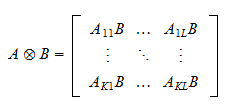wheredenotes the-th entry of.

In other words, the Kronecker productis a block matrix whose-th block is equal to the-th entry ofmultiplied by the matrix.

Note that, unlike the ordinary product between two matrices, the Kronecker product is defined regardless of the dimensions of the two matricesand.

## Examples

Although the concept is relatively simple, it is often beneficial to see several examples of Kronecker products.

Example DefineandThen,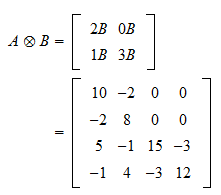Example DefineandThen,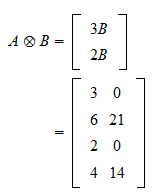Example Consider the two row vectorsandTheir Kronecker product is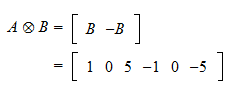Example Consider a row vectorand a column vectorThen, we have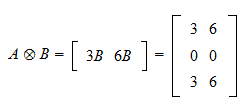Example Letbe theidentity matrix andany matrix. Then, their Kronecker product is the block matrixExample Letbe a scalar andany matrix. Then, computing their Kronecker product is the same as multiplyingby the scalar:Example Letbe any matrix anda scalar. Then,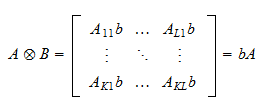where we have used the definition of multiplication of a matrix by a scalar.

## The Kronecker product is not commutative

The Kronecker product is not commutative, that is, in generalIt suffices to provide a single counterexample.

Example Define the matricesandThen, we have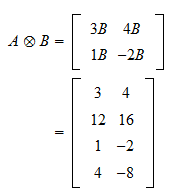and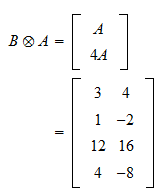## Properties

The many properties of Kronecker products will be discussed in the lecture on the Properties of the Kronecker product.

## Solved exercises

Below you can find some exercises with explained solutions.

### Exercise 1

LetandCompute.

Solution

We have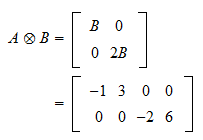### Exercise 2

LetandCompute.

Solution

We have